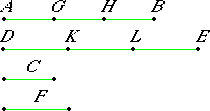# Proposition 15

Parts have the same ratio as their equimultiples.

Let AB be the same multiple of C that DE is of F.

I say that C is to F as AB is to DE.

Since AB is the same multiple of C that DE is of F, as many magnitudes as there are in AB equal to C, there are also in DE equal to F.

Divide AB into the magnitudes AG, GH, and HB equal to C, and divide DE into the magnitudes DK, KL, and LE equal to F. Then the number of the magnitudes AG, GH, and HB equals the number of the magnitudes DK, KL, and LE.V.7

And, since AG, GH, and HB equal one another, and DK, KL, and LE also equal one another, therefore AG is to DK as GH is to KL, and as HB is to LE.

Therefore one of the antecedents is to one of the consequents as the sum of the antecedents is to the sum of the consequents. Therefore AG is to DK as AB is to DE.

V.12

But AG equals C and DK equals F, therefore C is to F as AB is to DE.

Therefore, parts have the same ratio as their equimultiples.

Q.E.D.

## Guide

This proposition states that if n is any number and c and f any magnitudes of the same kind, then c : f = nc : nf.

This proposition is used in the next one and a few others in Books V, VI, and XIII.